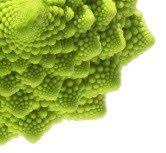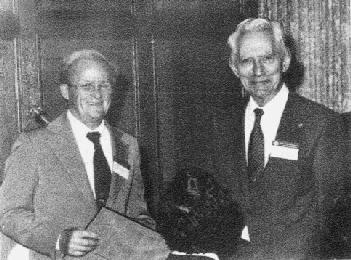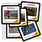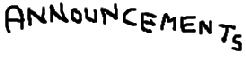## 582650 Information-Theoretic Modeling (5 cr) Department of Computer Science, University of Helsinki Fall 2014

An advanced course in the Algorithms and Machine Learning sub-programme. Students in other programmes, as well as students in mathematics and statistics are welcome too.

This course provides an introduction to information theory from the computer science perspective. Much of the course can be viewed as consequences of Shannon's central result known as the Noiseless Source Coding Theorem. The theoretical results will be illustrated by various practical data compression systems from Huffman coding to Rissanen's arithmetic coding. In order to demonstrate the wide applicability of information-theoretic concepts in Data Science, we discuss information-theoretic principles in statistical modeling, and especially the Minimum Description Length (MDL) principle.Jorma Rissanen, the inventor of the MDL principle (left) receiving a best paper award from Claude Shannon, the father of information theory.

Lecturer
Teemu Roos, A332, teemu.roos at cs.helsinki.fi
Teaching assistant
Jussi Määttä, jussi.maatta at helsinki.fi

Language: All course material and lectures will be in English.

Lectures: Period I: Sep 4–Oct 17, Wed & Fri 10–12 in C222.

Exercises: Sep 11–Oct 16, Thu 16–18 in C222.

Final exam: Oct 22, 9AM

The course is followed by a related project in Period II. It is recommended that you take both the course and the project. Link to: project.

Prerequisites:
• Elementary calculus (limits and convergence, convexity)
• Basic probability (random variables, conditional and joint distributions, expectation)
• Good programming skills (no specific language required)
Material:
• There is no course book. All the material will be contained in the lecture slides (below).
• A recommended source for complementary material is Cover & Thomas "Elements of Information Theory". There is an online version which you can access from the University network: online version
• Additional material categorized per theme is available below.

### Contents

The schedule below is tentative and subject to change.

Lecture 1. (Wed, Sep 3)
Introduction (attendance strongly recommended)
• introduction to the course • overview of contentsslidesexercises #1   |   example solutions (pdf)   |   solution code (zip)   |   link to Szpankowski's slides
Note: You should go through material under "Examples (with numbers)" starting on slide 105 by yourself.

Lecture 2. (Fri, Sep 5)
Mathematical Preliminaries
• calculus • probability • law of large numbers • Jensen's and Gibbs' inequalitiesslides

Lecture 3. (Wed, Sep 10)
Source Coding: Theory
• entropy and information • asymptotic equipartition property (AEP) • noiseless source coding theoremslidesexercises #2   |   example solutions (pdf)   |   solution code (zip)

Lecture 4. (Fri, Sep 12)
Channel Coding and Error Correction
• error correcting codes • noisy channel coding theorem • Hamming codesslides

Lecture 5. (Wed, Sep 17)
Source Coding: Practice I
• symbol codes • Kraft inequality • entropy coding • Shannon(-Fano) codesslidesexercises #3   |   example solutions (pdf)   |   solution code (zip)

Lecture 6. (Fri, Sep 19)
Source Coding: Practice II
• Huffman code • Shannon-Fano-Elias code • arithmetic codingslides

Lecture 7. (Wed, Sep 24)
Universal Source Coding I
• universal models • two-part codes | covered slides 1-14slidesexercises #4   |   example solutions (pdf)

Lecture 8. (Fri, Sep 26)
Universal Source Coding II
• mixture codes • normalized maximum likelihood | covered slides 15-46 (of Lecture 7)

Lecture 9. (Wed, Oct 1)
Universal Source Coding III
• prediction game • Bayes vs worst-case | covered slides 1-14slidesexercises #5   data200.txt   |   example solutions (pdf)   |   solution code (zip)

Lecture 10. (Fri, Oct 3)
MDL Principle: Introduction
• Occam's razor • model selection • old-style & modern MDL | covered slides 15-end (of Lecture 9)

Lecture 11. (Wed, Oct 8)
MDL Principle: Applications I
• encoding continuous data • differential entropy • Gaussian models | covered slides 1-22slides

Lecture 12. (Fri, Oct 10)
MDL Principle: Applications II
• multinomial models • histogram density estimation • clustering • Bayesian networks | slides 23-end (of Lecture 11)additional slidesexercises #6   survey.txt   splitcfg.py   |   example solutions (pdf)   |   solution code (zip)

Lecture 13. (Wed, Oct 15)
Further Topics
• Kolmogorov complexityslides

Lecture 14. (Fri, Oct 17)
Redundant Lecture
• summary of course contents • questions and answers before final exam • introduction to project

Here you can find recommended (but not required) additional material, categorized according to (our) lectures.
Lecture 1. Introduction
Claude Shannon - Father of the Information Age
University of California Television (UCTV), Youtube, 30min

Lecture 3. Source Coding: Theory
Cover & Thomas, Elements of Information Theory, Chapter 2
(see course folder, C127)

Claude E. Shannon, "A mathematical theory of communication", The Bell Systems Technical Journal, Vol. 27, pp. 379–423, 623–656, July-October, 1948.
a reprint (with corrections) from Bell Labs

Joaquin Candela, Probability, Information Theory and Bayesian Inference, on videolectures.net
• part 1: probability
• parts 2 & 3: entropy and information
• part 4: Bayesian inference

Lecture 4. Noisy Channel Coding
Information and Entropy
an MIT OpenCourseWare elementary level course
material on noise and errors (scroll down the page)

Lectures 5 & 6. Source Coding: Practice
Witten, Neal & Cleary, "Arithmetic coding for data compression", Communications of the ACM, Vol. 30, No 6, pp. 520–540, 1987.

Cover & Thomas, Elements of Information Theory, Chapter 5
(see course folder, C127)

Lelewer & Hirschberg, "Data compression", ACM Computing Surveys, Vol. 19, No. 3, pp. 261–296, 1987.

Ken Huffman, "Huffman algorithm", Huffman Coding Blog, Dec. 24, 2008.
a mostly informal blog entry written by David Huffman's nephew • relates Huffman code to mobiles (no, not cell phones)

David MacKay, Dasher: Information-Efficient Text Entry, Google Tech Talk, April 19, 2007.
dasher is a text-entry system based on arithmetic coding

David Solomon, Data Compression: The Complete Reference, Springer, 2004, 926 pages
the title says it all: a comprehensive reference; more recent editions available

Lectures 7 & 8 & 9: Universal Source Coding
Peter Grünwald, The Minimum Description Length Principle, MIT Press, 2007, 504 pages
• part 2: universal coding

Lectures 10 & 11 & 12: MDL Principle
Peter Grünwald, The Minimum Description Length Principle, MIT Press, 2007, 504 pages
• chapter 1: learning, regularity, and compression
PDF available

Peter Grünwald, MDL Tutorial, on videolectures.net

Jorma Rissanen, Minimum Description Length Principle, Scholarpedia, 3(8):6727.

Kontkanen & Myllymäki, "A linear-time algorithm for computing the multinomial stochastic complexity", Information Processing Letters, Vol. 103, No. 6, pp. 227–233, 2007.
a simple yet very useful result • quite complex proof

T. Silander, T. Roos, P. Kontkanen, and P. Myllymäki, (2008). Factorized NML criterion for learning Bayesian network structures, in Proceedings of the 4th European Workshop on Probabilistic Graphical Models (PGM-2008).
learns Bayesian network structures from data • uses the multinomial NML as a building block

Lecture 13: Kolmogorov Complexity (and Further Topics)
Li & Vitanyi, Kolmogorov Complexity and Its Applications, Springer, 1997.

R. Cilibrasi & P.M.B. Vitanyi, "Clustering by compression", IEEE Transactions on Information Theory, Vol. 51, pp. 1523–1545, 2005.
uses compression (gzip) to construct a universal similarity metric

R. Cilibrasi & P.B.B. Vitanyi, "The Google similarity distance", IEEE Transactions on Knowledge and Data Engineering, Vol. 19, pp. 370–383, 2007.
a follow-up on the previous • uses Google page counts to construct a semantic similarity metric

John L. Kelly, Jr., "A new interpretation of information rate", Bell Systems Technical Journal, Vol. 35, pp. 917–926, 1956.
we didn't cover this topic in the course • an argument for using logarithmic loss functions ⇒ PDF available

Video Coding Fundamentals & H.264, blog
not covered in the course
image coding
video codingSeptember 3 First lecture (C222); participation recommended. September 8, 4.15pm Distinguished lecture by Wojciech Szpankowski. Link to (PPT) slides. September 11 First exercise session (C222). Note changed room (was D122). September 12 Note the new instructions for exercises (pdf). October 2 We've set up a Piazza Q&A area for the course. All students have been sent an e-mail invitation to join. (If you didn't receive an invitation, send an email to Jussi.) You can ask any questions related to the lectures, homework problems etc. Anonymous queries are also allowed. October 22, 9.00AM Course exam (A111/B123/CK112). You are allowed to take a single double-sided A4-sized "cheat sheet" with your own hand-written (not photocopied from someone else) notes to the exam. A calculator is allowed but not necessary. November 14 Results of the course. (You need to have a CS department account to access.) November 21 Comments on the course exam and its grading October 29 C222 First "lecture" of the project. November 28, 4.00PM Separate exam in case you missed or failed the first one, B123. If you took part in the course, exercise points are valid for those who have completed at least 50% in case they improve your grade. Cheat sheet allowed (see above). December 17 Results of the separate exam. (You need to have a CS department account to access.) Exercise points were taken into account in cases where they resulted in a better grade.

 University of Helsinki | Department of Computer Science | Helsinki Institute for Information Technology